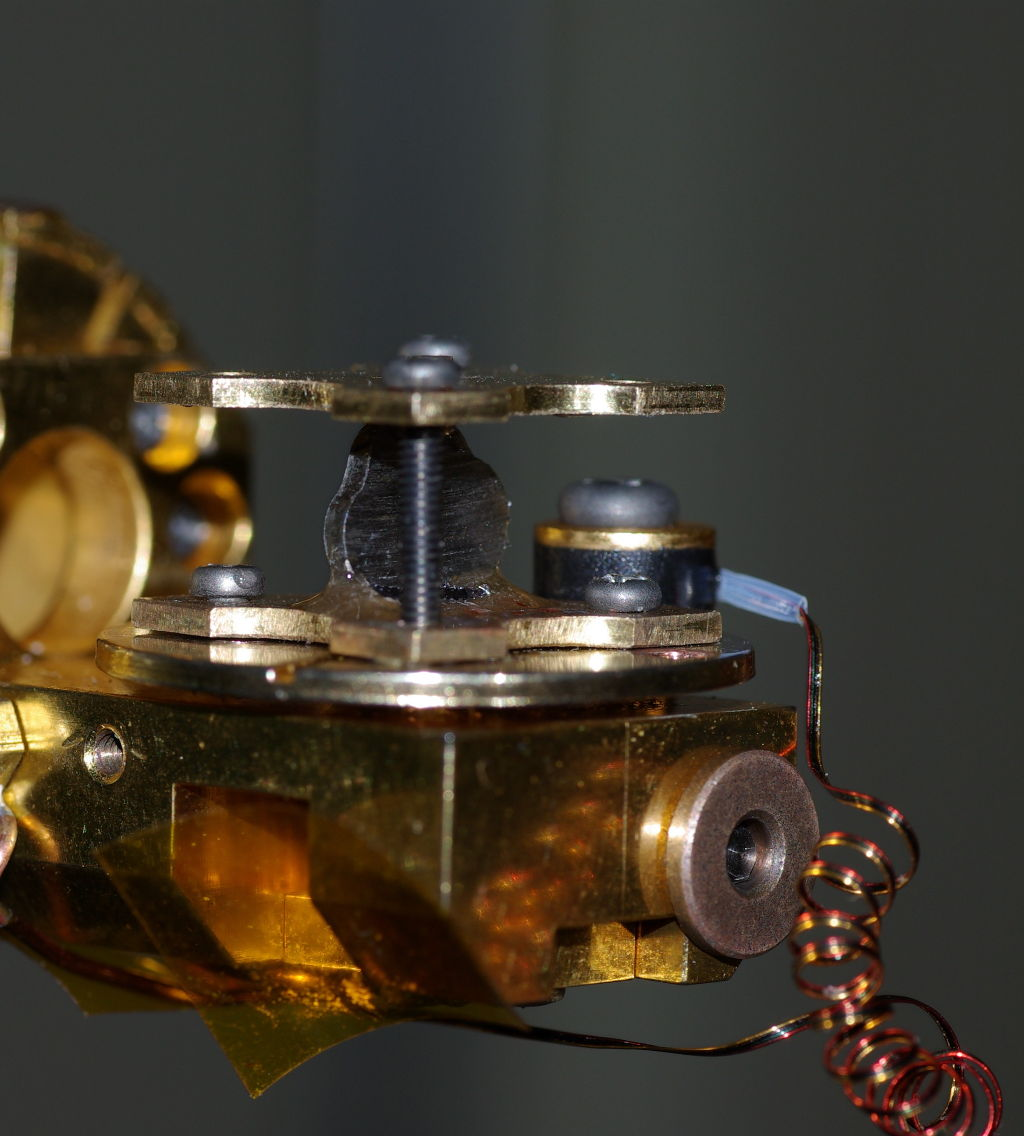# Magnetic Compton Scattering

1. ## First consider Charge Compton Scattering

We exploit the Compton effect to gain information on electron momentum distributions in crystal systems.

Compton scattering is the incoherent, inelastic scattering of a photon from a single electron, the scattered photon loses energy to the electron. The degree of energy loss is dependent on the angle of scattering. For a kinimatic analysis of the interaction the scattering must be within the impulse approximation (EI - Ef > Ebinding) and therefore treat all electrons as 'free' particles, we can ensure this is the case by selecting high energies.

As well as the 'shifted' Compton peak, the scattered spectrum exhibits Doppler broadening. This broadening is due to the finite momentum distribution of the electrons in the system. With the x-ray losing energy:$\hbar\omega = E' - E = \frac{p'^2}{2m} - \frac{p^2}{2m} = \frac{(\vec{p} + \hbar\vec{k})^2}{2m} - \frac{p^2}{2m} = \frac{\hbar k^2}{2m} + \frac{\hbar\vec{k}\cdot\vec{p}}{m} = \frac{\hbar^2k^2}{2m} + \frac{\hbar k}{m}p_z$

Where pz is the projection of the initial electron momentum along k, E is the initial energy and E' is the scattered photon energy.

For a system with only one momentum component the profile would be a Dirac delta function.

In a Compton scattering experiment we measure the 1D projection of the ground state electron momentum density n(p) of the system. Thus the different momentum components of characteristic electron shells within the atom result in the typical Charge Compton line shape resolved along the x-ray scattering vector.

The Compton profile, J(pz) is defined as:-$J(p_z) = \int\int n(\vec{p})dp_xdp_y$

The area under the Compton profile is equal to the number of electrons in the Wigner-Seitz cell.

.

## The Magnetic Compton Profile

Magnetic Compton Scattering (MCS) measures the 1D projection of the momentum density of the spin polarised electrons$[n_{\downarrow}(\vec{p}) - n_{\uparrow}(\vec{p})]$ in the system.

The magnetic Compton profile Jmag(pz) is defined as:$J_{mag}(p_z) = \int\int [n^{\uparrow}(\vec{p}) - n^{\downarrow}(\vec{p})]dp_xdp_y$$J_{mag}(p_z)$ can only be sampled in experiments in which the incident radiation is circularly polarised.

It follows from the description of charge Compton scattering that:-$\mu_{spin} = \int J_{mag}(p_z)dp_z$

How is Jmag(pz) accessed experimentally? and what about orbital magnetisation?

Firstly examine the cross section for spin polarised Compton scattering....

The relevant double differential cross-section for spin polarised Compton scattering is defined as :-$\frac{d^2\sigma}{d\Omega dE_2} = N\left(\frac{m}{\hbar k}\right)\left[\left(\frac{d\sigma}{d\Omega}\right)_{charge}J(p_z) + \left(\frac{d\sigma}{d\Omega}\right)_{mag}J_{spin}(p_z)\right]$

where$\left(\frac{d\sigma}{d\Omega}\right)_{charge} = \frac{1}{2}r_e^2(1+\text{cos}^2\theta)$$\left(\frac{d\sigma}{d\Omega}\right)_{mag} = -r_e^2\tau P_2(1 + \text{cos}^2)\theta)(\hat{q}\text{cos}\theta + \hat{q}')\cdot\vec{m}$

Experimentally this equates to either:-

• Using a phase plate to reverse the helicity of the radiation
• OR flipping the samples magnetisation with an appropriate magnetic field.

The latter is favoured since the use and optimisation of a phase plate at high energies is non-trivial.

Either case results in the measurement of a flipping, or asymmetry ratio R, defined as:-$R = \left(\frac{I_{\uparrow} - I_{\downarrow}}{I_{\uparrow} + I_{\downarrow}}\right) = \frac{\int\limits_0^\infty\left(\frac{d\sigma}{d\Omega}\right)_{mag}J_{spin}(p_z)dE_2}{\int\limits_0^\infty\left(\frac{d\sigma}{d\Omega}\right)_{charge}J_{spin}(p_z)dE_2}$

##### Here muspin is the total spin moment.

In magnetic materials only a small percentage of electrons are unpaired and responsible for the magnetism. Therefore charge Compton scattering dominates i.e. R ~ 1-2% of the total measured intensity (in systems with a large spin moment e.g. Fe, Gd)

In other ferro / ferri magnets R can be <0.5% or less !!

This means to optimise the experiment, use back scattering geometry where the magnetic term in the DD cross section is a maximum.

Use High energies to enhance the measured R factor, increase detector resolution and ensure that the sample bulk is measured.

Use more than one detector. ... and wait !